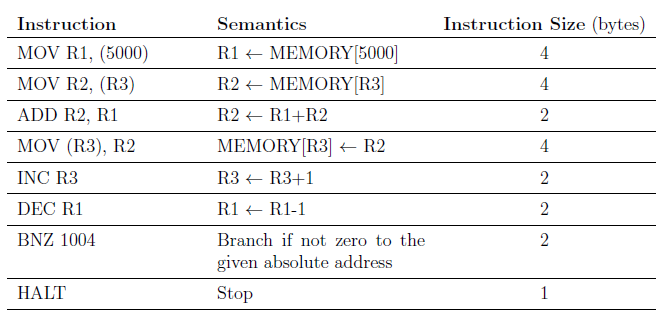Related Articles
GATE | GATE CS 2021 | Set 1 | Question 65
• Last Updated : 06 Apr, 2021

Consider the following instruction sequence where registers R1,R2 and R3 are general purpose and MEMORY[X] denotes the content at the memory location X.Assume that the content of the memory location 5000 is 10, and the content of the register R3 is 3000. The content of each of the memory locations from 3000 to 3010 is 50. The instruction sequence starts from the memory location 1000. All the numbers are in decimal format. Assume that the memory is byte addressable.

After the execution of the program, the content of memory location 3010 is ____________ .
(A) 50
(B) 60
(C) 51
(D) 49

Answer: (A)

Explanation: The content of each of the memory locations from 3000 to 3010 is 50.

Given, code modifies given array element,
60, 59, 58, 57, 56, 55, 54, 53, 52, 51, 50

Hence, 50 is the correct answer.

Quiz of this QuestionMy Personal Notes arrow_drop_up